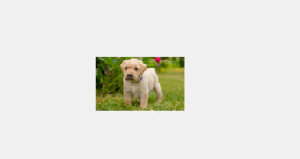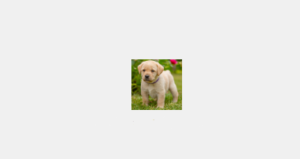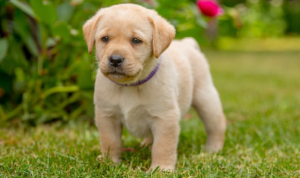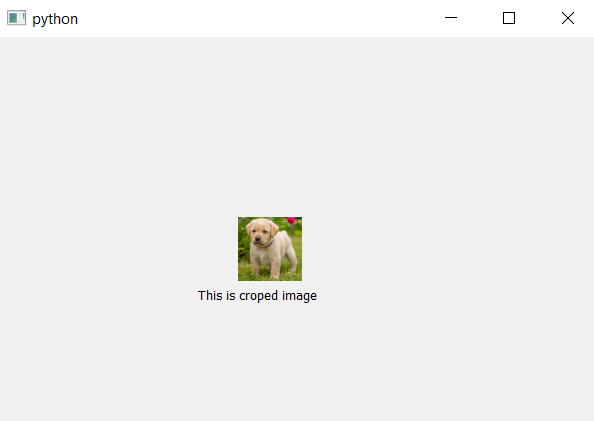# PyQt5 – How to get cropped square image from rectangular image ?

• Last Updated : 26 Mar, 2020

In this article, we will see how to display only cropped square image from any rectangular image with any width and height i.eAttention geek! Strengthen your foundations with the Python Programming Foundation Course and learn the basics.

To begin with, your interview preparations Enhance your Data Structures concepts with the Python DS Course. And to begin with your Machine Learning Journey, join the Machine Learning - Basic Level Course

In order to do so we have to do the following steps :

2. Crop image to make it square
3. Mask it and make perfect square from it using Painter
4. Convert it back to pixmap image

Code –

For this original image below is the code :`# importing libraries``from` `PyQt5.QtCore ``import` `*` `from` `PyQt5.QtGui ``import` `*` `from` `PyQt5.QtWidgets ``import` `*`` ` `# function to alter image``def` `mask_image(imgdata, imgtype ``=``'png'``, size ``=` `64``):`` ` `    ``# Load image``    ``image ``=` `QImage.fromData(imgdata, imgtype)`` ` `    ``# convert image to 32-bit ARGB (adds an alpha ``    ``# channel ie transparency factor):``    ``image.convertToFormat(QImage.Format_ARGB32)`` ` `    ``# Crop image to a square:``    ``imgsize ``=` `min``(image.width(), image.height())``    ``rect ``=` `QRect(``        ``(image.width() ``-` `imgsize) ``/` `2``,``        ``(image.height() ``-` `imgsize) ``/` `2``,``        ``imgsize,``        ``imgsize,``    ``)``    ``image ``=` `image.copy(rect)`` ` `    ``# Create the output image with the ``    ``# same dimensions and an alpha channel``    ``# and make it completely transparent:``    ``out_img ``=` `QImage(imgsize, imgsize, QImage.Format_ARGB32)``    ``out_img.fill(Qt.transparent)`` ` `    ``# Create a texture brush and paint a circle``    ``# with the original image onto``    ``# the output image:``    ``brush ``=` `QBrush(image)`` ` `    ``# Paint the output image``    ``painter ``=` `QPainter(out_img)``    ``painter.setBrush(brush)`` ` `    ``# Don't draw an outline``    ``painter.setPen(Qt.NoPen)`` ` `    ``# drawing square``    ``painter.drawRect(``0``, ``0``, imgsize, imgsize)`` ` `    ``# closing painter event``    ``painter.end()`` ` `    ``# Convert the image to a pixmap and rescale it.``    ``pr ``=` `QWindow().devicePixelRatio()``    ``pm ``=` `QPixmap.fromImage(out_img)``    ``pm.setDevicePixelRatio(pr)``    ``size ``*``=` `pr``    ``pm ``=` `pm.scaled(size, size, Qt.KeepAspectRatio, ``                               ``Qt.SmoothTransformation)`` ` `    ``# return back the pixmap data``    ``return` `pm`` ` ` ` `class` `Window(QWidget):`` ` `    ``"""Simple window that shows our masked image and text label."""``    ``def` `__init__(``self``):``        ``super``().__init__()`` ` `        ``# setting up the geometry``        ``self``.setGeometry(``100``, ``100``, ``600``, ``400``)`` ` `        ``# image path``        ``imgpath ``=` `"image.png"`` ` `        ``# loading image``        ``imgdata ``=` `open``(imgpath, ``'rb'``).read()`` ` `        ``# calling the function``        ``pixmap ``=` `mask_image(imgdata)`` ` `        ``# creating label``        ``self``.ilabel ``=` `QLabel(``self``)`` ` `        ``# putting image on label``        ``self``.ilabel.setPixmap(pixmap)`` ` `        ``# moving the label``        ``self``.ilabel.move(``240``, ``180``)`` ` `        ``# another label to put text``        ``self``.tlabel ``=` `QLabel(``'This is croped image'``, ``self``)``        ``self``.tlabel.move(``200``, ``250``)`` ` ` ` ` ` `# main function``if` `__name__ ``=``=` `'__main__'``:``    ``import` `sys``    ``from` `PyQt5.QtWidgets ``import` `QApplication`` ` `    ``# app created``    ``app ``=` `QApplication(sys.argv)``    ``w ``=` `Window()``    ``w.show()`` ` `    ``# begin the app``    ``sys.exit(app.exec_())`

Output :My Personal Notes arrow_drop_up